Courses

# GOC & Aromaticity - 1

## 20 Questions MCQ Test GATE Chemistry Mock Test Series 2020 | GOC & Aromaticity - 1

Description
This mock test of GOC & Aromaticity - 1 for Chemistry helps you for every Chemistry entrance exam. This contains 20 Multiple Choice Questions for Chemistry GOC & Aromaticity - 1 (mcq) to study with solutions a complete question bank. The solved questions answers in this GOC & Aromaticity - 1 quiz give you a good mix of easy questions and tough questions. Chemistry students definitely take this GOC & Aromaticity - 1 exercise for a better result in the exam. You can find other GOC & Aromaticity - 1 extra questions, long questions & short questions for Chemistry on EduRev as well by searching above.
QUESTION: 1

### Which is more nucleophilic than the other of the following compounds?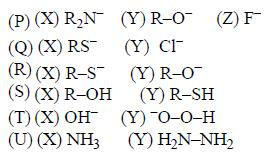Solution:

Nucleophilicity increases with the increase in the size of atom in same period and decreases with the increase in electronegativity.

QUESTION: 2

### The correct order of the pKa values for the conjugate acids of heterocyclic compounds given below is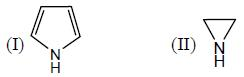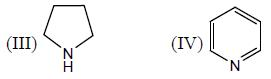Solution: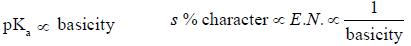Basicity: Electron pair donating ability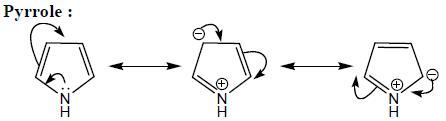Delocalised lone pair present on nitrogen whcih have lowest basicity.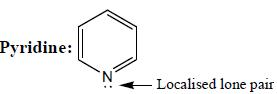Pyridine have poor basic character. In pyridine, lone pair present on the nitrogen atom are perpendicular
to the orthogonal orbital, containing sp2 hybrid orbital.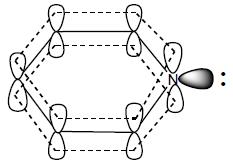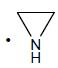(Aziridine) having more s% character than pyrrolidine. so, pyrrolidine is more basic than Aziridine).

So, correct order of pka value.

III>II<IV>I

QUESTION: 3

### Pick out the incorrect statements

Solution:

Resonance involve the displacement of lone pair and double bonds of the molecules without changing the position of the atoms. Tautomerization involve the change in position of lone pair and double bond with atom

QUESTION: 4

The correct order of pKa for the following compounds is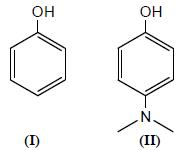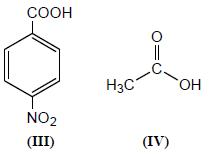Solution: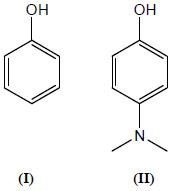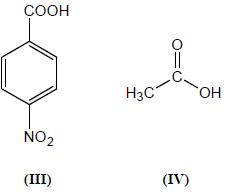• Electron withdrawing group incereses the acidic character
• Electron donating group decreses the acidic behaviour
•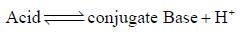• Higher the Ka, lower will be pka, higher will be acidity => acidic behaviour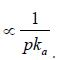• Nitro Benzoic acid is more acidic as compared to acetic acid, which in turn is more acidic as compared to phenol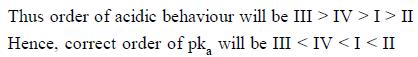QUESTION: 5

Consider the following reaction sequence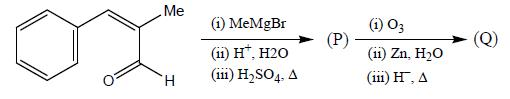Product (P) and (Q) are made to react with sodium amide to yield R and S respectively. Identify the correct statement.

Solution: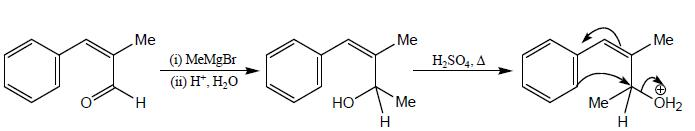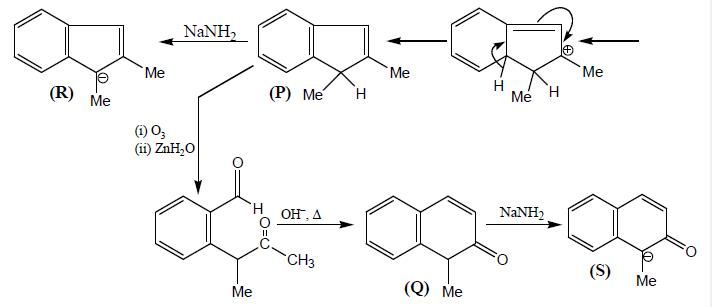QUESTION: 6

Consider the bonds marked as (A), (B), (C) and (D) in the following structures.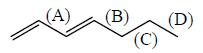What is the correct order of their homolytic bond dissociation energies

Solution:

If the intermediate free radical which is formed via homolytic cleavage is more stable, the bond breakage is more feasible, and thus bond dissociation energy will be less. Since (C) forms, stabilized conjugated free radical, its homolytic bond dissociation energy will be least.  Followed by the same approach, the order will be  A > B > D > C. Hence, correct option is (c).

QUESTION: 7

The correct order of dipole moment in pyrrole, thiophene and furan respectively is

Solution:

There are three major factor contributing to the overall dipole moments

(i) The σ bond dipole associated with C-0 and C—N bonds

(ii) The π bond dipole associated with delocalisation of π electrons from the heteroatom to the ring.

(iii) The dipole moment associated with the unshared electron pair (for 0) or N—H bond (for N)

• All these factors have a greater moment towards rather than away from the heteroatom for furan thanfor pyrrok.
• For pyrrok, the C-N π dipole should be larger and the N—H moment in the opposite direction from furam .These two factor account for the reversal in the direction of overall dipole moment.

In furan and thiophene the dipole moment is influenced by resonance in the same way as in the pyrrok. However, the effect of resonance is not sufficiently enough to exceed the effect of heteroatom electronega-tivity. Thus, the direction of dipole moment will be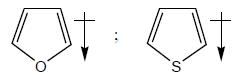QUESTION: 8

The rotational energy barrier along 1-2 bond will be the lowest in

Solution:

As we know that rotation around double bond require breakage of laterally overlapped bond. Hence, it is not energetically favourable to rotate around double bond as compared to single bond.

In structure given in option (b) and (d). There is considerable double bond character around 1-2 bond due to delocalisation.

(a) will remain in energetically favourable uncharged form, because there no driving force like aromaticity to push it in charged form.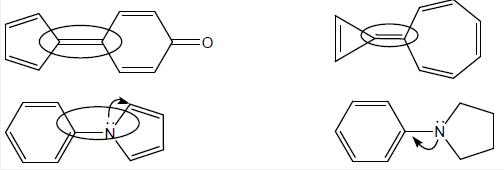Lone pair not available for delocalization into benzene ring..

QUESTION: 9

The compound with lowest heat of combustion will be

Solution:

More stable olefin will have lesser heat of hydrogenation and lesser heat of combustion.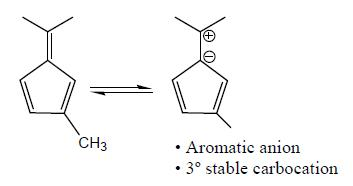*Answer can only contain numeric values
QUESTION: 10

Consider the following reactions,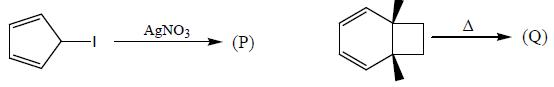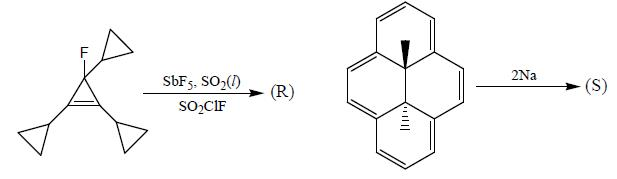A boy is said to choose one entity from product P, Q, R and S. What is the probability that the chosen compound will be antiaromatic.

Solution:

It is found that among P,Q, R and S,P and S are antiaromatic i.e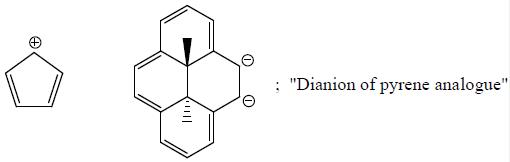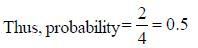QUESTION: 11

Given compounds A,B,C and D are subjected to IR spectrum analysis. compounds which will show minimum and maximum vco streching frequency in IR respectively will be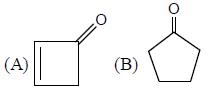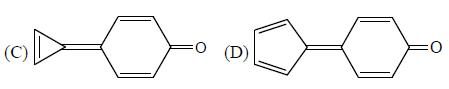Solution:

vco, stretching frequency ∝ strength of bond. Thus, the species, in which there is more delocalisation, C-0 bond have considerable single bond character and thus have lower value of stretching frequency.

Hence, among A, B, C and D, B will have maximum value of vca,  Since, its — C=0 bond is intact. On the other hand, C is an aromatic compound thus, it must have charge separation, which leads to &localisation. Which in turn makes C = 0 bond, considerable single bond character. Thus, C will have minimum value stretching frequency.

QUESTION: 12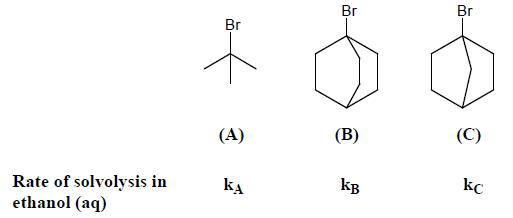If polarity of solvent is sufficiently increased, then what will happen?

Solution:

Rate of sololysis in aqueous ethanol (weak nucleophile) will proceed preferentially by SN 1 mechanism. since, rate of SN 1 reaction ∝ stability of carbocation. Here, stability order of carbocation will be in the order of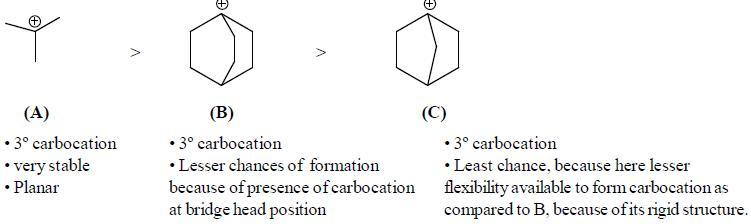QUESTION: 13

Consider the following sequence of reaction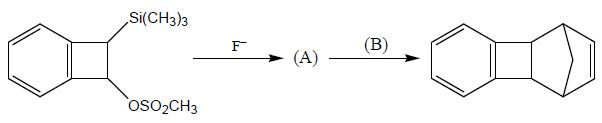B on reaction with Na in presence of FeCl2 gives a well known organometallic compound C. Identify the correct statement about above reaction sequence.

Solution: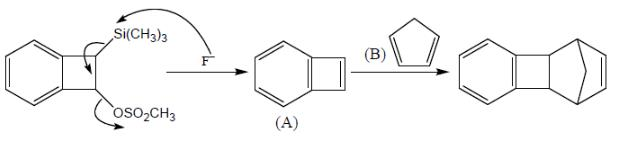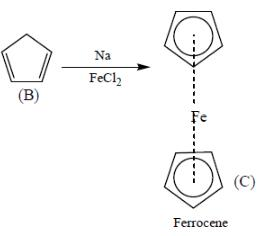From the above reaction sequence, it is clear that A and B not an aromatic compound, whicle C which is ferrocene is a well know aromatic compound

QUESTION: 14

Among the pairs given below, identify the most acidic compound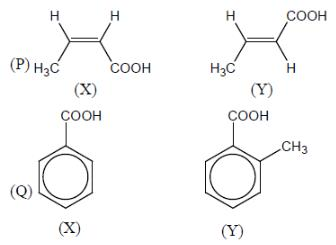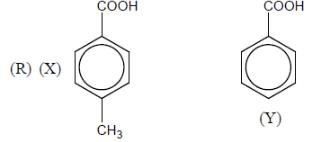Solution: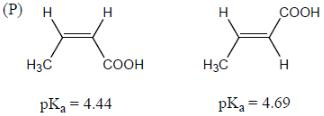Generally, cis acid are more acidic than trans acid, if group are small.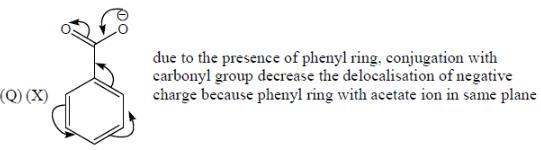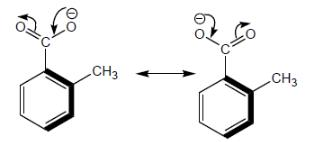(Y) due to the presence of methyl group ortho to carboxylate ion. which are perpendicular to phenyl ring. So. carboxylate group not in conjugate with phenyl ring. Hence. carboxylate negative ion having more conjugation. So. (Y) is more acidic in nature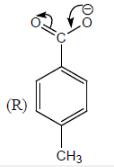Presence of electron releasing group, decreases the stability of negative charged conjugate base. So, less acidic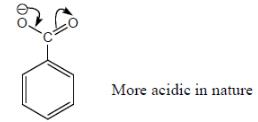*Answer can only contain numeric values
QUESTION: 15

The number of anti-aromatic systems present among the following compounds is/are _____________(Answer should be an integer)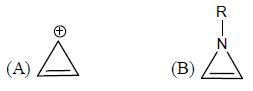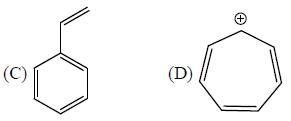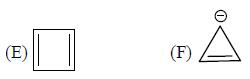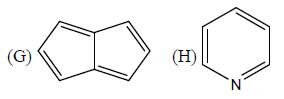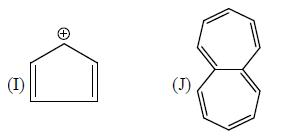Solution:

B, E, F, and I are anti-aromatic in nature

*Answer can only contain numeric values
QUESTION: 16

Among the following, how many are aromatic in nature?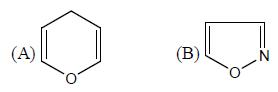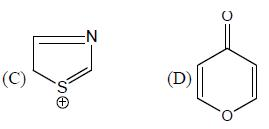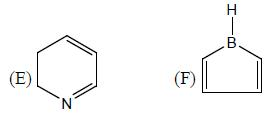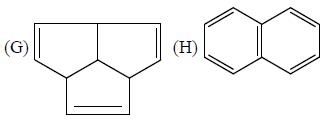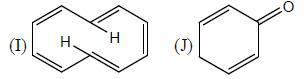Solution:

B, D, H aromatic in nature

*Answer can only contain numeric values
QUESTION: 17

The number of aromatic compound among following will be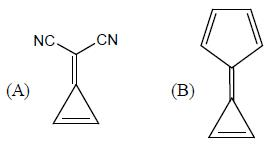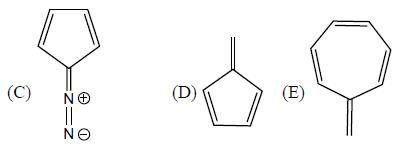Solution: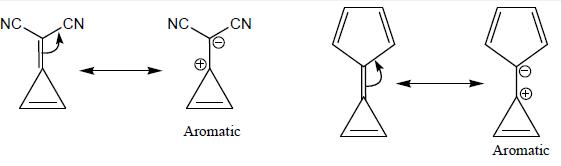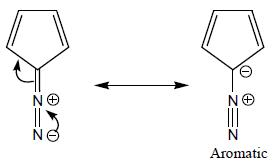No such driving force for delecalization present in (d) and (e)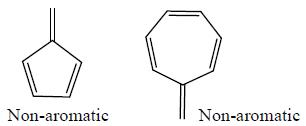QUESTION: 18

What is the sequence of reagents that will accomplish the synthesis of the following aromatic amine from benzene?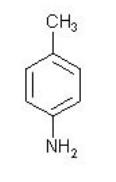Solution:
*Answer can only contain numeric values
QUESTION: 19

In the following compounds, how many pairs of compounds having correct order w.r.t mentioned properties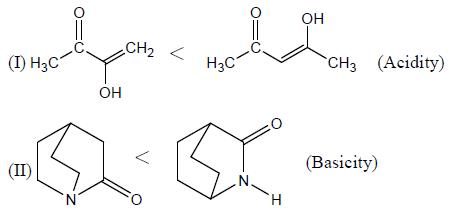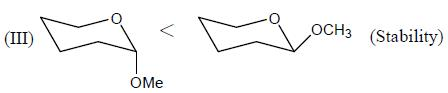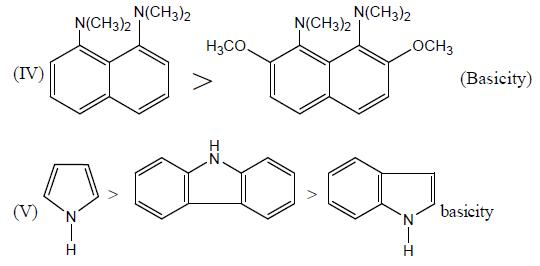Solution: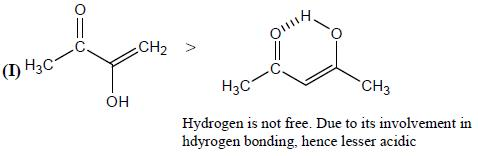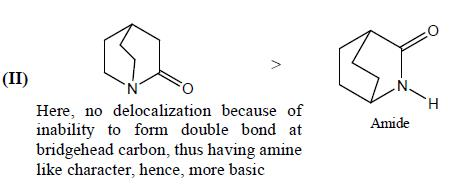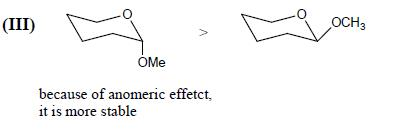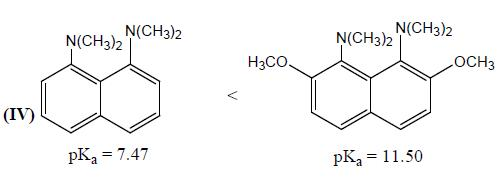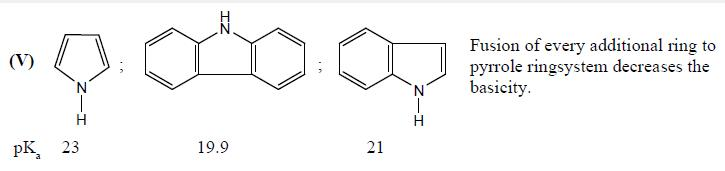*Answer can only contain numeric values
QUESTION: 20

In the followig compounds, how many compounds having more pKa value relative to the H2o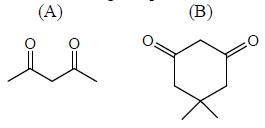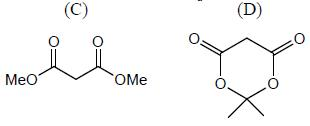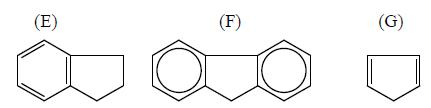Solution:

H2O = pKa value 15.74 (more pka value than H20 means less acidic than H20)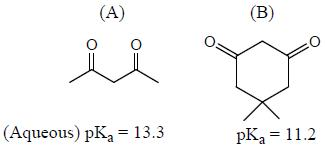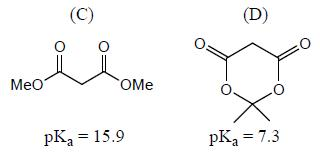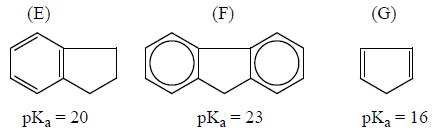Compounds C,E,F,G having more pKa value than H20